# Check if there is a root to leaf path with the given sequence

In this article, we are going to see how to check whether there is any root to leaf path with the given path sequence?
Submitted by Radib Kar, on August 20, 2020

Here, we are going to see given a binary tree if there exist any root to leaf path which matches with the given sequence. For example, in the below tree,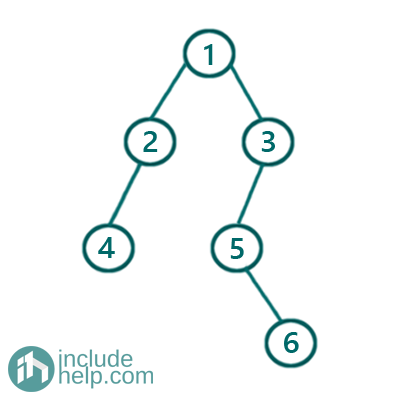Say the path sequence is [1, 3, 5, 6] then, there is a root to leaf path which matches with this sequence. The path sequence is shown below,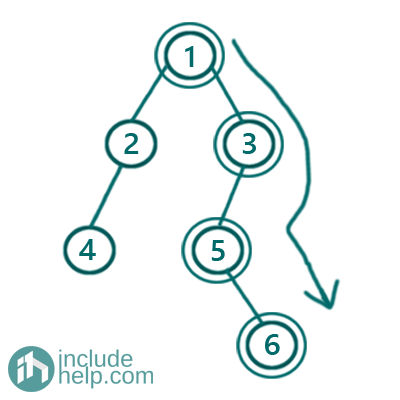But if the given path sequence is [1,4] or [1,4, 6] then we are unable to find any root to leaf path which matches with the given sequences.

Solution:

1. Brute-force:
One way is we can store each root->leaf paths and search if any path matches with the given sequence. But this brute force method is not at all entertained as it will be time-consuming and also requires adequate storage which should not be entertained.
2. Efficient approach:
The efficient  approach is like below using recursion:
```Function isValidSequenceRecur(TreeNode* node, int current_index vector& path):
1)  Base cases:
a)  If the current node is NULL before
the path ends then it's false
b)  if the path ends and the current node is a leaf node
then we found the sequence, so return true
c) if path ends, but the current node is not leaf
node then return false
2)  Check if thecurrent node value matches with
current Path index value, path[current_index]
If it doesn't match immediately return false
Otherwise
Check if rest of the path belongs to any of the subtrees
End Function
```

Below is the implementation of the above approach.

```#include <bits/stdc++.h>
using namespace std;

// tree node is defined
class TreeNode {
public:
int val;
TreeNode* left;
TreeNode* right;
TreeNode(int data)
{
val = data;
left = NULL;
right = NULL;
}
};

bool isValidSequenceRecur(TreeNode* root, int index, vector<int>& arr)
{
//base cases
//1. if root is NULL before the path ends then it's false
if (!root)
return false;

//2. if the path ends and its a leaf node then we found the sequence
if (!root->left && !root->right && index == arr.size() - 1 && root->val == arr[index])
return true;

//3. if path ends, but it's not leaf node then  return false
if (index == arr.size())
return false;

//if rootr value matches with current path index value recur for both of its subtrees
//if any subtree return true then we can assure that we found the sequence
if (root->val == arr[index])
return isValidSequenceRecur(root->left, index + 1, arr) || isValidSequenceRecur(root->right, index + 1, arr);
else //if root value doesn't match with current path index value the return false
return false;
}

bool isValidSequence(TreeNode* root, vector<int>& arr)
{
return isValidSequenceRecur(root, 0, arr);
}

int main()
{
//building the tree like example 1
TreeNode* root = new TreeNode(1);

root->left = new TreeNode(2);
root->right = new TreeNode(3);
root->left->left = new TreeNode(4);
root->right->left = new TreeNode(5);
root->right->left->right = new TreeNode(6);

vector<int> path1{ 1, 2, 4 };
vector<int> path2{ 1, 3, 4, 5 };

if (isValidSequence(root, path1))
cout << "Path sequence 1 is found in the tree\n";
else

if (isValidSequence(root, path2))
cout << "Path sequence 2 is found in the tree\n";
else

return 0;
}
```

Output:

```Path sequence 1 is found in the tree
```

Dry run with the examples:

1) Where given sequence matches with a root to leaf path

Path=[1, 2, 4]

Tree: as shown as in the example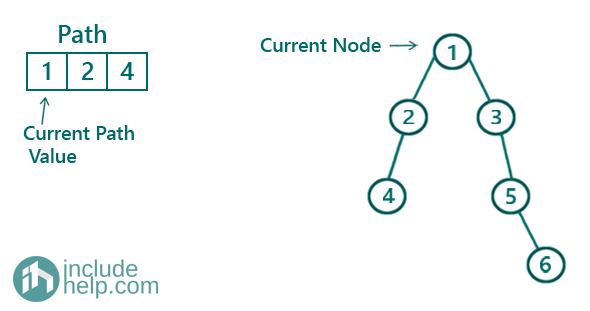So, both the current path value and current node value matches and thus we need to find the rest of the path sequence in any of the subtrees.

Recur for the left subtree first,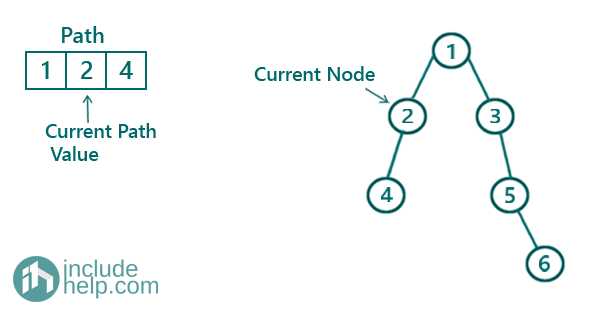Again, both the current path value and current node value matches and thus we need to find the rest of the path sequence in any of the subtrees of the current node.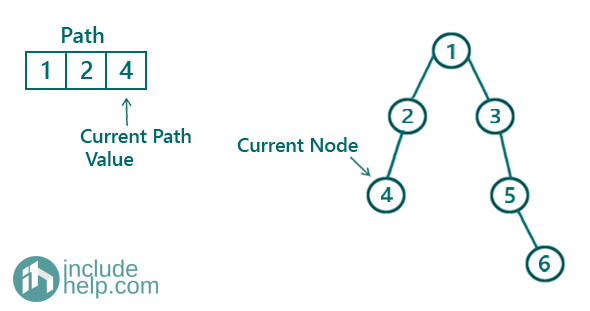Now, it meets the3 base condition b, i.e., the path ends and Current node is a leaf node then we found the sequence, so return true.

Thus we found the sequence and the path is [1, 2, 4]

2) Where given sequence doesn't match with any root to leaf path

Path=[1, 3, 4, 5]

Tree: as shown as in the example

So, both the current path value and current node value matches and thus we need to find the rest of the path sequence in any of the subtrees.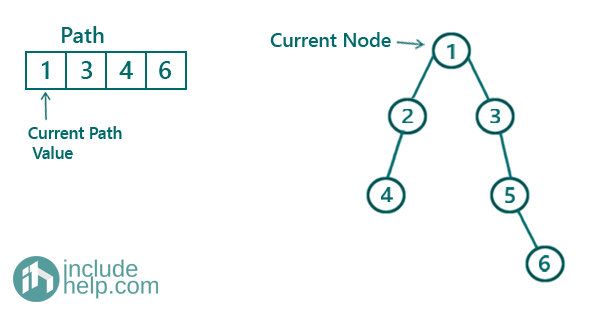So, both the current path value and current node value matches and thus we need to find the rest of the path sequence in any of the subtrees.

Recur for the left subtree first,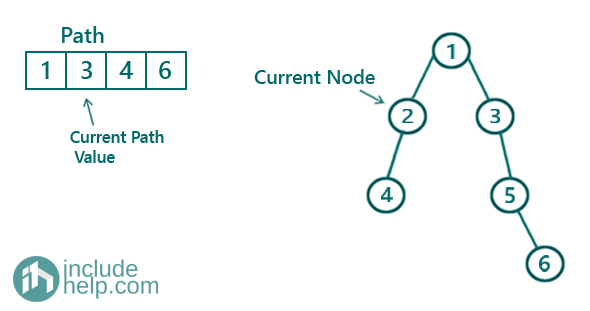Here, the path value doesn't match with the current node value and does it returns false. So control goes back to the previous calling function and it finds left subtree giving false, so it goes for the right subtree & same current path value.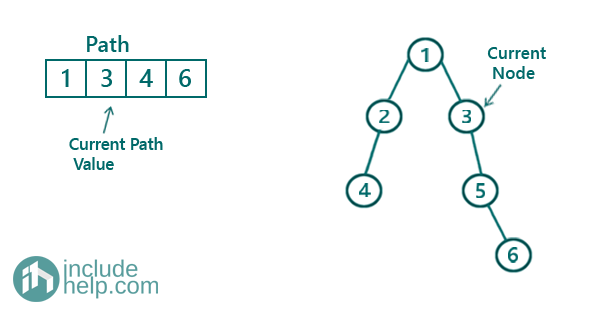Again, here the current node value matches with the current index value and we find if any subtrees of the current node contain the rest of the path. But the left subtree of the current node is 5 which doesn’t match with the next path value(4) and the right subtree is NULL. So There is no path which matches with the given sequence.

What's New

Top Interview Coding Problems/Challenges!

Languages: » C » C++ » C++ STL » Java » Data Structure » C#.Net » Android » Kotlin » SQL
Web Technologies: » PHP » Python » JavaScript » CSS » Ajax » Node.js » Web programming/HTML
Solved programs: » C » C++ » DS » Java » C#
Aptitude que. & ans.: » C » C++ » Java » DBMS
Interview que. & ans.: » C » Embedded C » Java » SEO » HR
CS Subjects: » CS Basics » O.S. » Networks » DBMS » Embedded Systems » Cloud Computing
» Machine learning » CS Organizations » Linux » DOS
More: » Articles » Puzzles » News/Updates